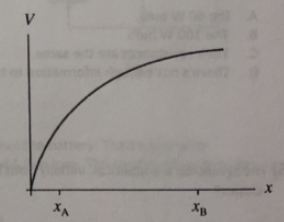# Problem: At what point is the electric field stronger?A. at xAB. at xBC. The field is the same strength at both.D. There's not enough information to tell.

###### FREE Expert Solution

Electric field:

$\overline{){\mathbf{E}}{\mathbf{=}}{\mathbf{-}}\frac{\mathbf{∆}\mathbf{V}}{\mathbf{∆}\mathbf{x}}}$

The above expression shows that the magnitude of the slope is equal to the magnitude of the slope.

81% (217 ratings)###### Problem DetailsAt what point is the electric field stronger?

A. at xA

B. at xB

C. The field is the same strength at both.

D. There's not enough information to tell.